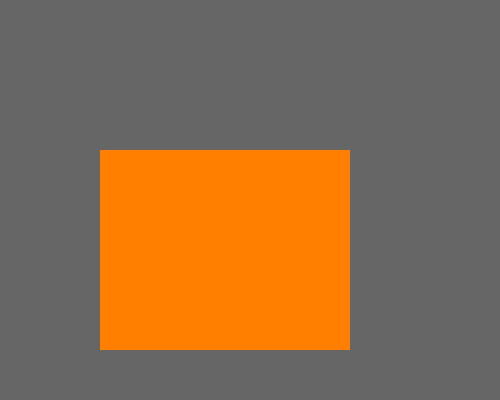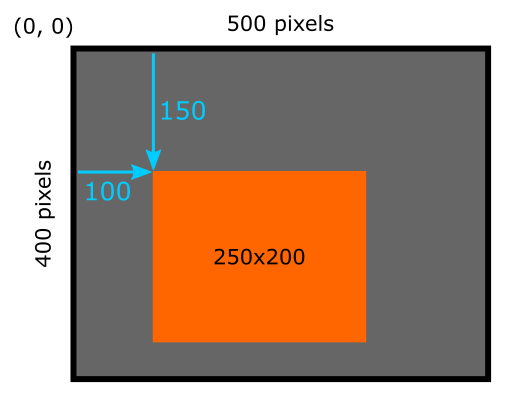# Simple image in generativepy

Martin McBride, 2021-11-07
Tags generativepy tutorial rectangle draw setup
Categories generativepy generativepy tutorialIn this article we will create a very simple vector image using generativepy. The image looks like this:generativepy can also be used to create bitmap images, NumPy images, 3D images, and videos. These will be covered in later tutorials, but the basic process is similar in all cases.

## The code

Here is the code to draw the image above:

from generativepy.drawing import make_image, setup
from generativepy.color import Color
from generativepy.geometry import Rectangle

def draw(ctx, pixel_width, pixel_height, frame_no, frame_count):

setup(ctx, pixel_width, pixel_height, background=Color(0.4))

color = Color(1, 0.5, 0)

Rectangle(ctx).of_corner_size((100, 150), 250, 200).fill(color)

make_image("rectangle.png", draw, 500, 400)


This code is available on github in tutorial/getting-started/rectangle.py.

We will now look at this in more detail.

## Basic structure

The basic structure of the code is:

• A user-defined function draw that does the drawing.
• A call to make_image. This function controls the process of creating an image and saving it to a file. We pass in the draw function to control the image content.

This basic structure is used throughout generativepy.

For vector images, like this one, generativepy uses the Pycairo library. In fact, as we will see, ctx is a Pycairo drawing context.

## make_image

The make_image function, from the drawing module, is the main function that handles creating vector images.

The basic parameters are:

• The filename of the output PNG file.
• A draw function that does the actual drawing.
• The width and height of the image in pixels.

The draw function is a function that we define ourselves to perform the drawing. It doesn't have to be called draw - you can call it whatever you like, provided you pass its name into make_image. It must have the correct parameters.

## draw function

draw accepts 5 parameters:

• ctx is a Pycairo context. This is like a virtual drawing surface that you can draw on in code. Whatever you draw will appear on the final image.
• pixel_width and pixel_height are the width and height of the image in pixels. These are the values that we passed into make_image.
• frame_no and frame_count are only used when you want to create image sequences (for videos), so we can ignore them here.

generativepy uses Pycairo as its drawing library. Pycairo has an extensive set of drawing functions that you can use to draw on the context, but generativepy also provides some extra functions that are useful for generative art. You can mix and match these functions - that is especially useful if you want to use some advanced feature of Pycairo that generativepy doesn't directly support. It is usually best to use the generativepy functions if possible, as they act at a slightly higher level.

Our draw function calls the setup function to set up the drawing area, then draws a rectangle.

## setup function

The setup function isn't mandatory, but it does some useful things so you will often want to call it.

setup accepts 3 required parameters - the ctx, pixel_width and pixel_height that were passed in to the draw function. It has some optional parameters that do various things.

In this case, we are setting the background parameter to Color(0.4) which sets the background colour to a mid grey (it sets r, g and b to 40%). We define the colour using the color module of generativepy.

We also create another colour, Color(1, 0.5, 0) for use later on. This is 100% red, 50% green and 0% blue, which is an orange colour.

## Drawing a rectangle

We use a Rectangle object to draw a rectangle, like this:

Rectangle(ctx).of_corner_size((100, 150), 250, 200).fill(color)


All shapes are drawn using the same pattern:

• Declare a shape object, for example Rectangle(ctx). The context is passed in at this stage.
• An of_xxx function is used to define the shape. In this case, of_corner_size defines a rectangle from the position of its corner, and its width and height. The corner is specified using an (x, y) tuple.
• A drawing function is then used to actually draw the shape. In this case, we use fill that fills the shape with the supplied orange colour.

## Coordinate system

By default, the size and position of shapes we draw using generativepy are measured in pixels, based on the size of the image we are creating.

• The origin of the coordinate system (0, 0) is at the top left of the image.
• x values increase from left to right.
• y values increase as you move down the image.

In our case:

• Our image is 500 by 400 pixels.
• The orange rectangle is 250 by 200 pixels.
• The position of the rectangle (that is, the position of its top-left corner) is at pixel position (100, 150).

This diagram illustrates the coordinates of the rectangle:## Experiments

The example place is a good place to start if you want to experiment with other shape objects, which all work in a similar way.

If you found this article useful, you might be interested in the book Computer Graphics in Python or other books by the same author.

#### Popular tags

2d arrays abstract data type alignment and angle animation arange arc array arrays behavioural pattern bezier curve built-in function callable object chain circle classes clipping close closure cmyk colour combinations comparison operator comprehension context context manager conversion count creational pattern data science data types design pattern device space dictionary drawing duck typing efficiency ellipse else encryption enumerate fill filter font font style for loop function function composition function plot functools game development generativepy tutorial generator geometry gif global variable gradient greyscale higher order function hsl html image image processing imagesurface immutable object in operator index inner function input installing iter iterable iterator itertools join l system lambda function len line linear gradient linspace list list comprehension logical operator lru_cache magic method mandelbrot mandelbrot set map matplotlib monad mutability named parameter numeric python numpy object open operator optimisation optional parameter or pandas partial application path pattern permutations polygon positional parameter print pure function python standard library radial gradient range recipes rectangle recursion reduce repeat rgb rotation roundrect scaling scipy sector segment sequence setup shape singleton slice slicing sound spirograph sprite square str stream string stroke structural pattern subpath symmetric encryption template text text metrics tinkerbell fractal transform translation transparency triangle truthy value tuple turtle unpacking user space vectorisation webserver website while loop zip